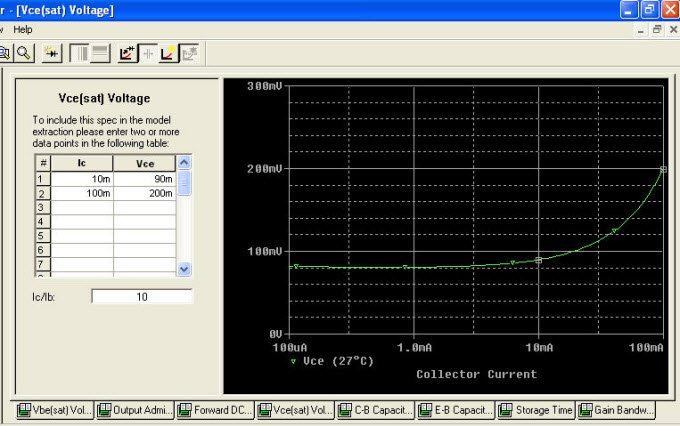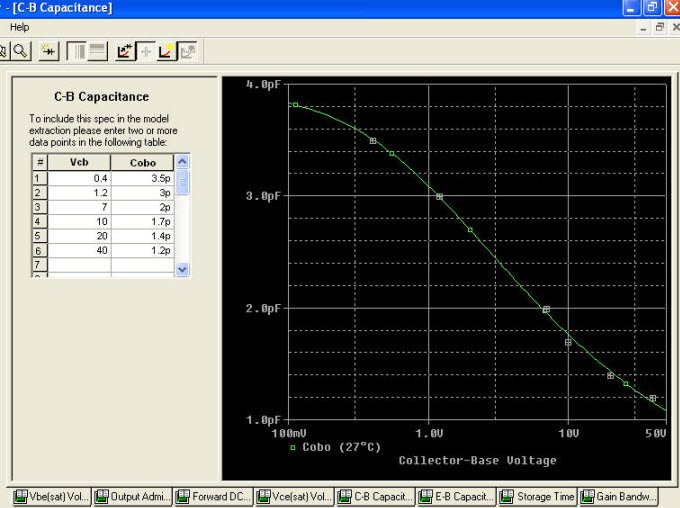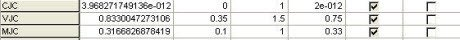# SPICE modeling of a BJT from Datasheet

Let’s fix data and continue.Add data of Vce(sat) versus Ic:and get BR=0.608, IKR=2.152, ISC= 13.68 fA, NC=1.80, e RC=0.86 Ohm:as usual, fix the data and see capacitances graph:input Cob data and get the curve:extracted data are CJC = 3.968pF, VJC = 0.833V, MJC = 0.316:input data for Cib and we get:from which we derive CJE = 6.808pF, VJE = 1.319 V, MJE = 0.477:Posted in PSpice Modeling from Datasheet and tagged , , , .